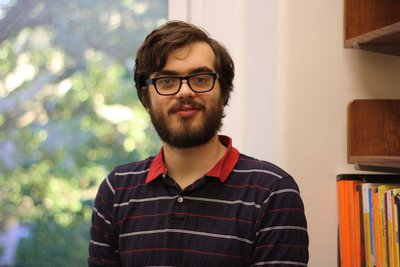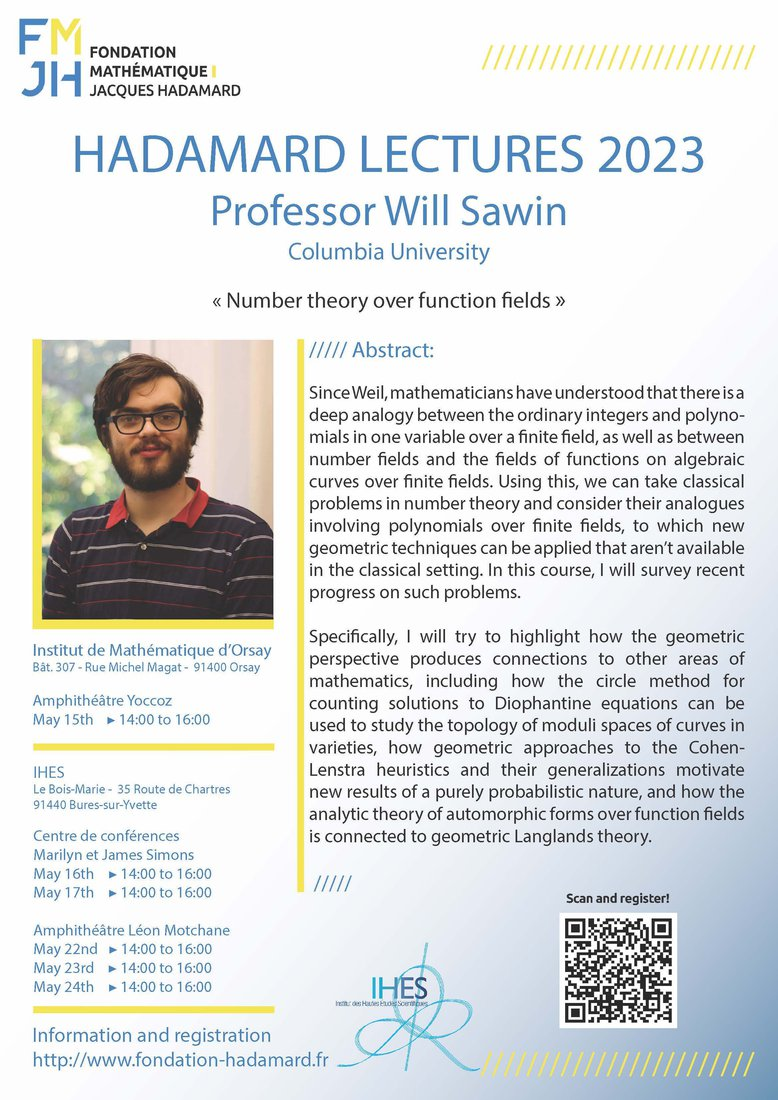# Will Sawin

(Columbia University in New York)

The winner of the SASTRA Ramanujan 2021 prize
whose research interests include applications of
étale cohomology to analytic number theory.

## May 15th at Institut de Mathématique d'Orsay May 16th, 17th, 22nd, 23rd and 24th at IHES

### "Number theory over function fields"

Abstract: Since Weil, mathematicians have understood that there is a deep analogy between the ordinary integers and polynomials in one variable over a finite field, as well as between number fields and the fields of functions on algebraic curves over finite fields. Using this, we can take classical problems in number theory and consider their analogues involving polynomials over finite fields, to which new geometric techniques can be applied that aren't available in the classical setting. In this course, I will survey recent progress on such problems.

Specifically, I will try to highlight how the geometric perspective produces connections to other areas of mathematics, including how the circle method for counting solutions to Diophantine equations can be used to study the topology of moduli spaces of curves in varieties, how geometric approaches to the Cohen-Lenstra heuristics and their generalizations motivate new results of a purely probabilistic nature, and how the analytic theory of automorphic forms over function fields is connected to geometric Langlands theory.

***  Registration is mandatory ***